# Berkeley Science Books

Home

Mathematical Modeling
and
Computational Calculus I

CWT Vol 1 - Constant Velocity Motion

CWT Vol 2 - Newton's Apple

CWT Vol 3 - Nature's Favorite Functions

CWT Vol 4 - Good Vibrations - Fourier Analysis and the LaPlace Transform

New in 2007 - CWT - College Edition

New in 2006 - FREEMAT

The Wave Equation

Airplane Simulator

Planetary Motion

Maxwell's Equations

Relativity

GR

Berkeley Science Books
St. Petersburg, FL
727-389-7011
send email# Calculus Without Tears

## The Finite Difference Method

### 1. Introduction

The Basic Paradigm of Mathematical Physics and Engineering

Since the time of Newton the basic paradigm of mathematical physics and engineering has been the following:
1. Understand the physical laws governing the phenomena being studied.
2. Develop a differential equation model of the process.
3. Solve the differential equations. Ay, there's the rub.
4. Analyze the results

The Need for Computational Calculus

The problem has always been step 3, as most differential equations do not have analytic solutions.

Thus, the student takes a year of calculus in high school, and then a series of courses in college consisting of differential calculus, integral calculus, multi-variable calculus, vector calculus, and culminating in a course in differential equations. So far no physical systems have been analyzed, and the student is never told that most problems can't be solved using analytic calculus. The student proceeds to a course in Fourier analysis and the Laplace transform, followed by a course in linear systems analysis, and finally, a few weeks before graduation, real systems, linear ones that is, can be analyzed analytically.

If a student takes an upper division or graduate course in dynamics, or astrorphysics, or material science, or electronics, or Maxwell's equations, or fluid dynamics, non-linear systems will be encountered, and he/she will be introduced to computational calculus, which is how most physics and engineering problems are solved today. See, for example, the online course Dynamics of Physical Systems, https://www.youtube.com/playlist?list=PLD074EEC1EBEFAEA5 , where computational calculus is introduced when it's time to solve problems, in lecture 20.

But, here is the thing, computational calculus is trivially easy and can be taught in high school. It doesn't require years of study, Euler's method, used in MMCC I, and its extension to partial differential equations, the finite difference method used in MMCC II, can be fully explained in an hour ! Then, its just a matter of practice, which is what we do in the MMCC books.

The Finite Difference Method

The finite difference method (FDM), applied to ordinary differential equations, is Euler's method, the method used in MMCC I. Given a differential equation Euler's method substitutes an algebraic expression, essentially the expression defining the derivative, for the derivative in the differential equation, the substitution is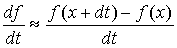This is just our old favorite, velocity, df/dt, = distance f(x+dt) - f(x), divided by time, dt. The resulting algebraic equation is then used to compute, using arithmetic operations only, solutions to the original ordinary differential equation.

The FDM applied to a partial differential equation uses the following substitutions for the partial spatial derivatives in the differential equation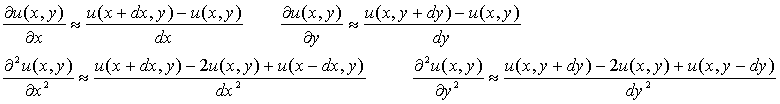And, the resulting algebraic equation is then used to compute, using arithmetic operations only, solutions to the original partial differential equation.

The salient properties of the FDM are that it is easy to learn, intuitively clear, works the same for every problem, and it is very powerful and can be used to solve a wide variety of problems.

The Program's the Thing

We follow the basic paradigm for each project in the book, starting with the physical laws, and giving a derivation of the differential equation model in baby steps, striving for intuitive clarity and transparency. At that point the FDM substitutions are made in the model, and a MATLAB/OCTAVE/FREEMAT program is written to implement the computations. So, finally, exerything in the model is made explicit, in terms of additions, subtractions, multiplications, and divisions. Writing a program enables the student to see exactly how each component of the model affects other components and how it contributes to the total solution. In addition, by varying model and system parameters, the process can be studied for a variety of model characteristics, initial conditions and disturbance functions. The program gives the student a sense of complete mastery of the mathematical analysis of the physical process.

MATLAB has excellent built-in graphing features, and the graphs below, with one exception, did not require any programming at all beyond computing the data to be graphed.

### 2. Heat Transfer

Physical Laws

The physical law governing heat transfer is Fourier's Heat Transfer Law, which is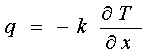Differential Equation Model of Heat Transfer

The differential equation model is derived from Fourier's Heat Transfer Law, for heat transfer in 2-D the model is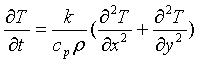Notes: Derivation

Projects

Now we make the FDM substitutions into the model, and compute.
Notes: Computation

1-D heat transfer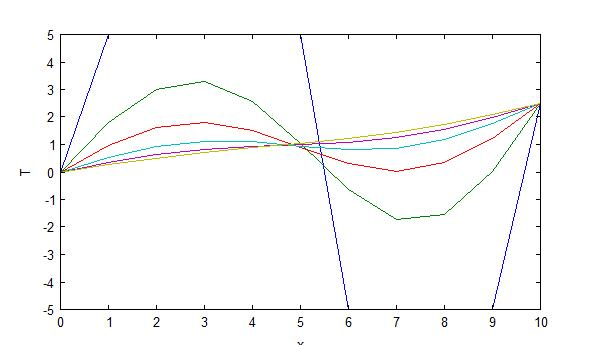...2-D heat transfer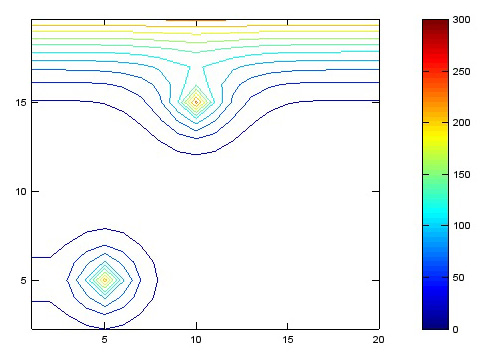...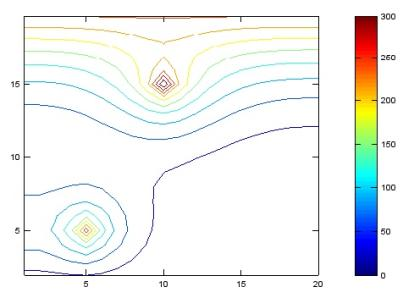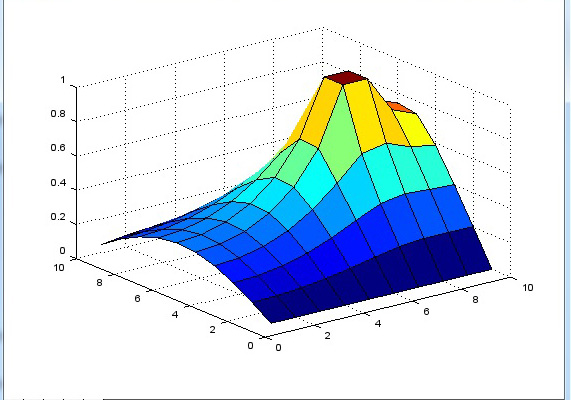### 3. The Wave Equation

Physical Laws

The wave equation can be derived from a number of different physical laws, in this chapter it is derived from Newton's 2nd Law of Motion, F = MA. It is also derived from Maxwell's equations in the chapter on Maxwell's equations.

Differential Equation Model of the Wave Equation

The differential equation model for the wave equation in 2-D is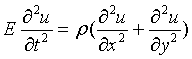Projects

1-D wave equation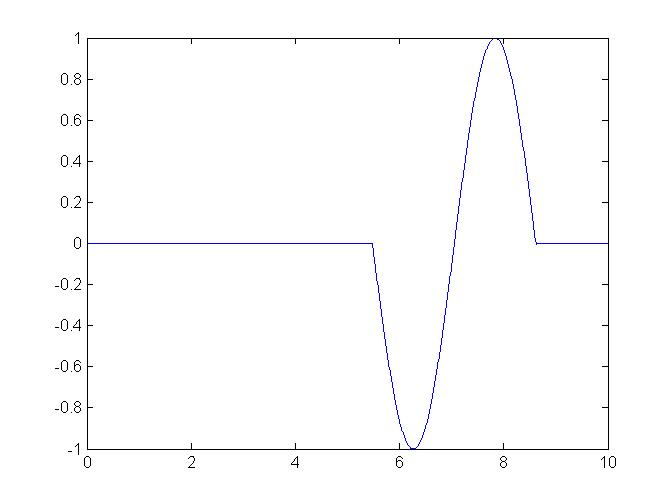...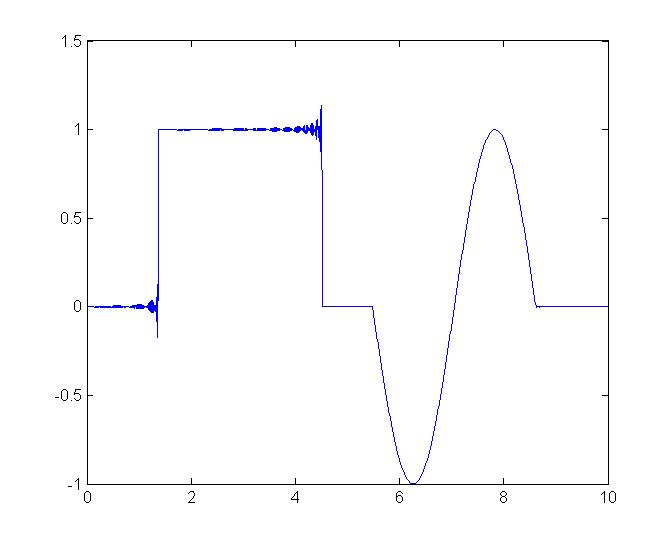2-D wave equation...### 4. Stress and Strain

Physical Laws

The stress and strain model is derived from Newton's 2nd Law of Motion, and Hoooke's Law. Hooke's Law in 1-D is the familiar spring law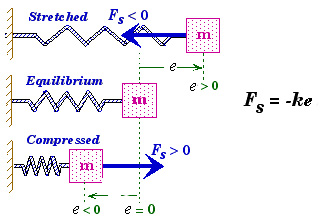Things get a little more interesting in 2-D and 3-D, where there are shear strains and stresses. Hooke's Law in 2-D takes the form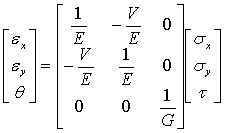where E = Young’s modulus
V = Poisson’s ratio
G = shear modulus
εx, εy, σx, σy denoting normal strains and stresses
θ, τ denoting shear strain and stress.

Differential Equation Model for Stress and Strain

The equilibrium model for stress is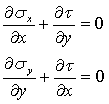Making the substitutions from Hooke's 2-D law gives the model in terms of displacements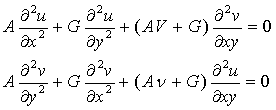Projects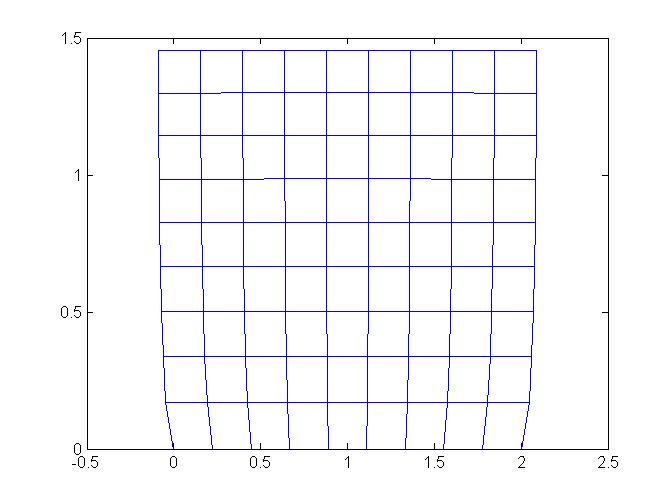...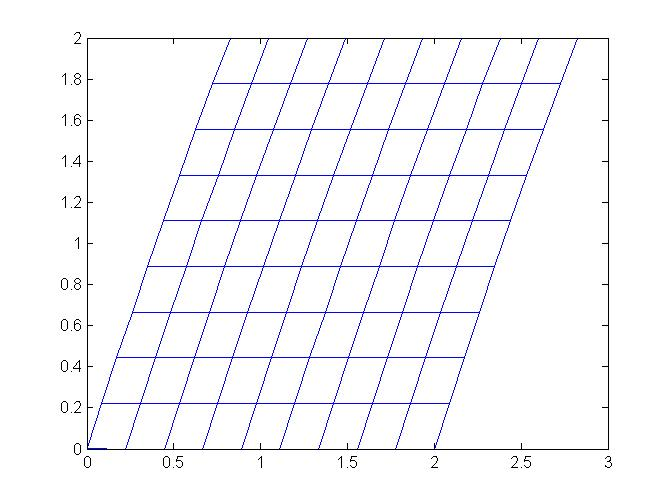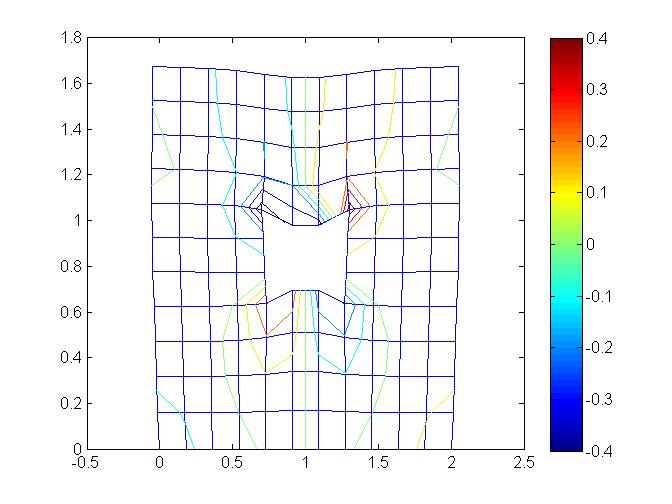...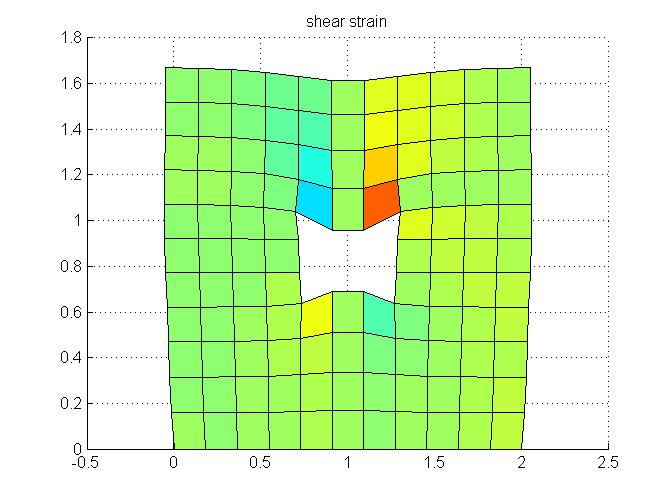### 5. Fluid Dynamics

Physical Laws

The differential equation model for fluid motion, the Navier-Stokes equations, are derived from Newton's 2nd Law of Motion, the Reynold's Transport Theorem, which let's us apply Newton's 2nd law of motion to a control volume of fluid, and Newton's Law of Viscosity, which states that the shear stress is proportional to shear strain rate for fluids.

Differential Equation Model for Fluid Motion

The derivation of the Navier-Stokes equations from the physical laws is given in complete detail, the Navier-Stokes equations are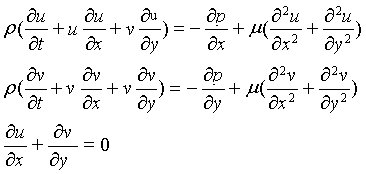Notes: The momentum equations

Projects

Cavity flow ..................................................................................... channel flow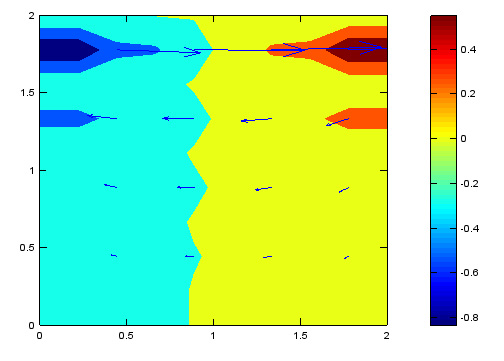...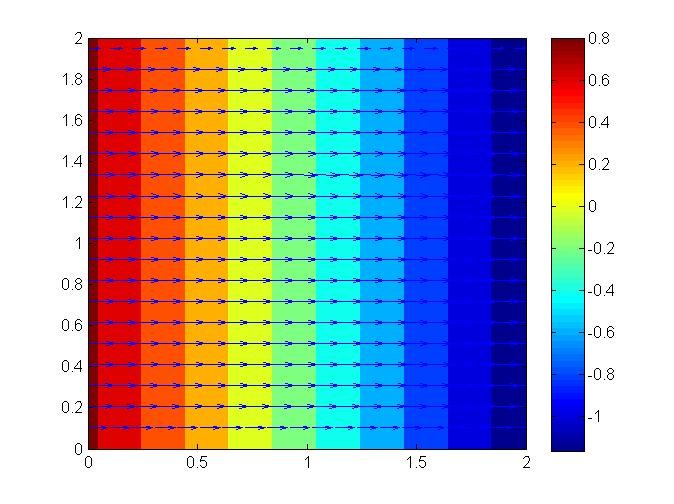Flow over backward step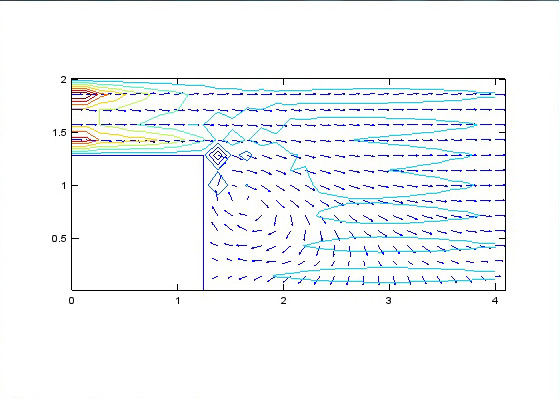### 6. Maxwell's Equations and Electromagnetic Radiation

Physical Laws

Maxwell's equations follow directly from four simple experiments, their derivation is given in complete detail in the book.
Notes: Derivation

Differential Equation Model for Electromagnetic Radiation

Maxwell's 3rd and 4th equations in differential form are the model for electromagnetic radation, in 2-D they are in expanded form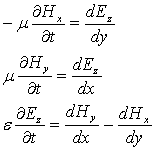Projects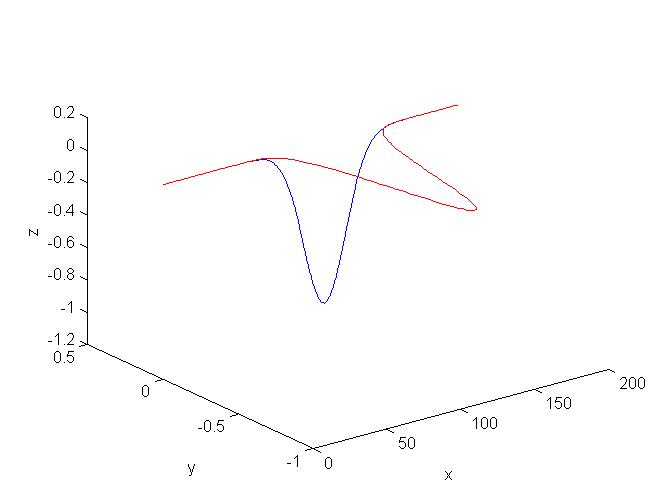...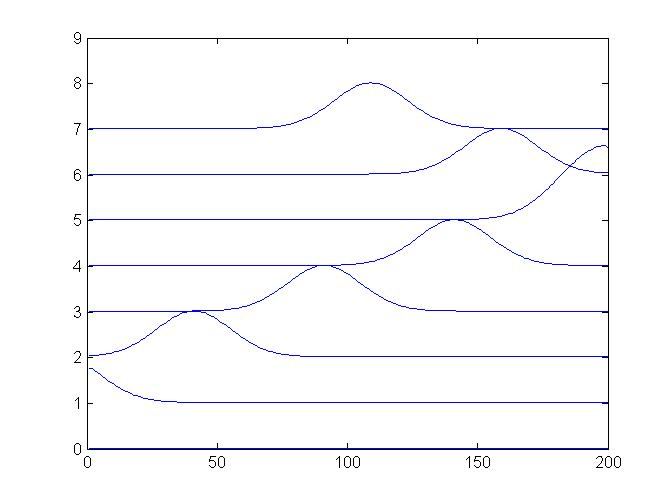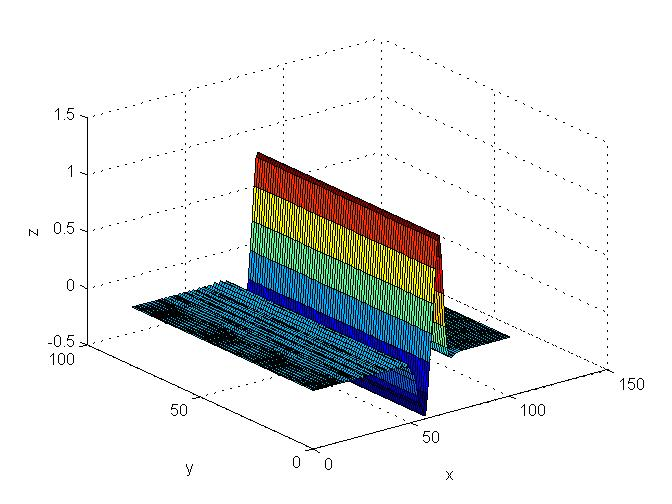...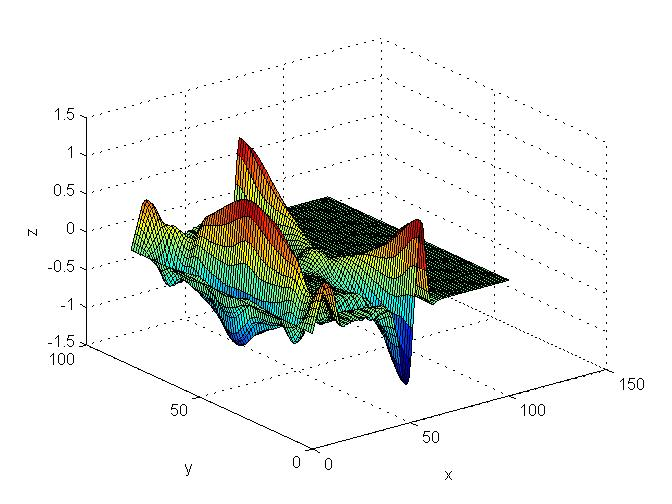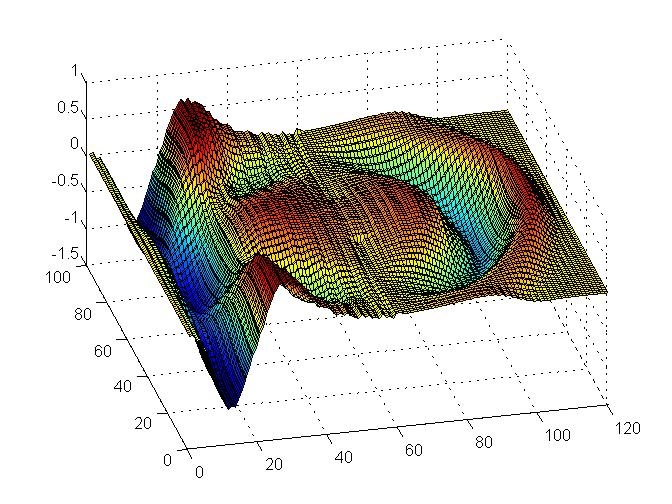...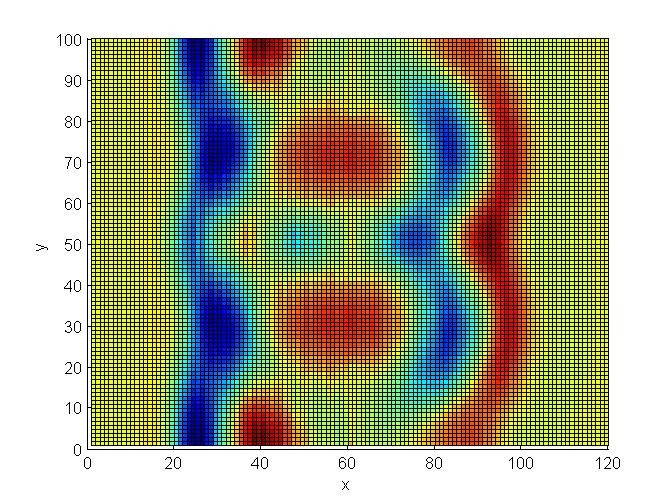### 7. Consistency, Stability, and Convergence

Von Neumann's Method for Stability Analysis

Examples